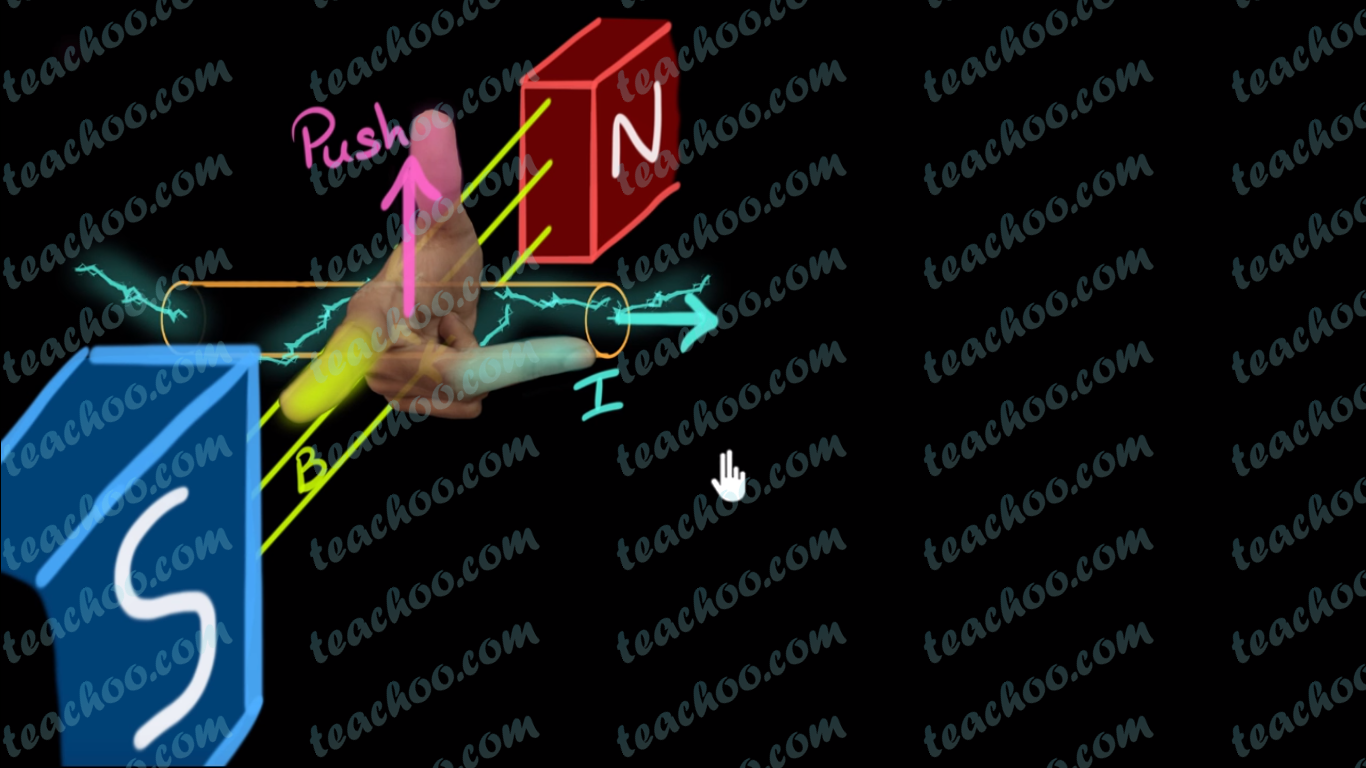Concepts

Class 10
Chapter 13 Class 10 - Magnetic Effects of Electric Current (Term 2)

In Electromagnetic Induction, we saw that we can induce electric current by

• Moving wire in a fixed Magnetic Field
• Changing magnetic field keeping Wire fixed

But, how to find Direction of Induced Current?

We find Direction of Induced Current Using Fleming's Right Hand Rule

On our Right Hand,

We put our Thumb, Index finger and Middle Finger perpendicular to each other as shown below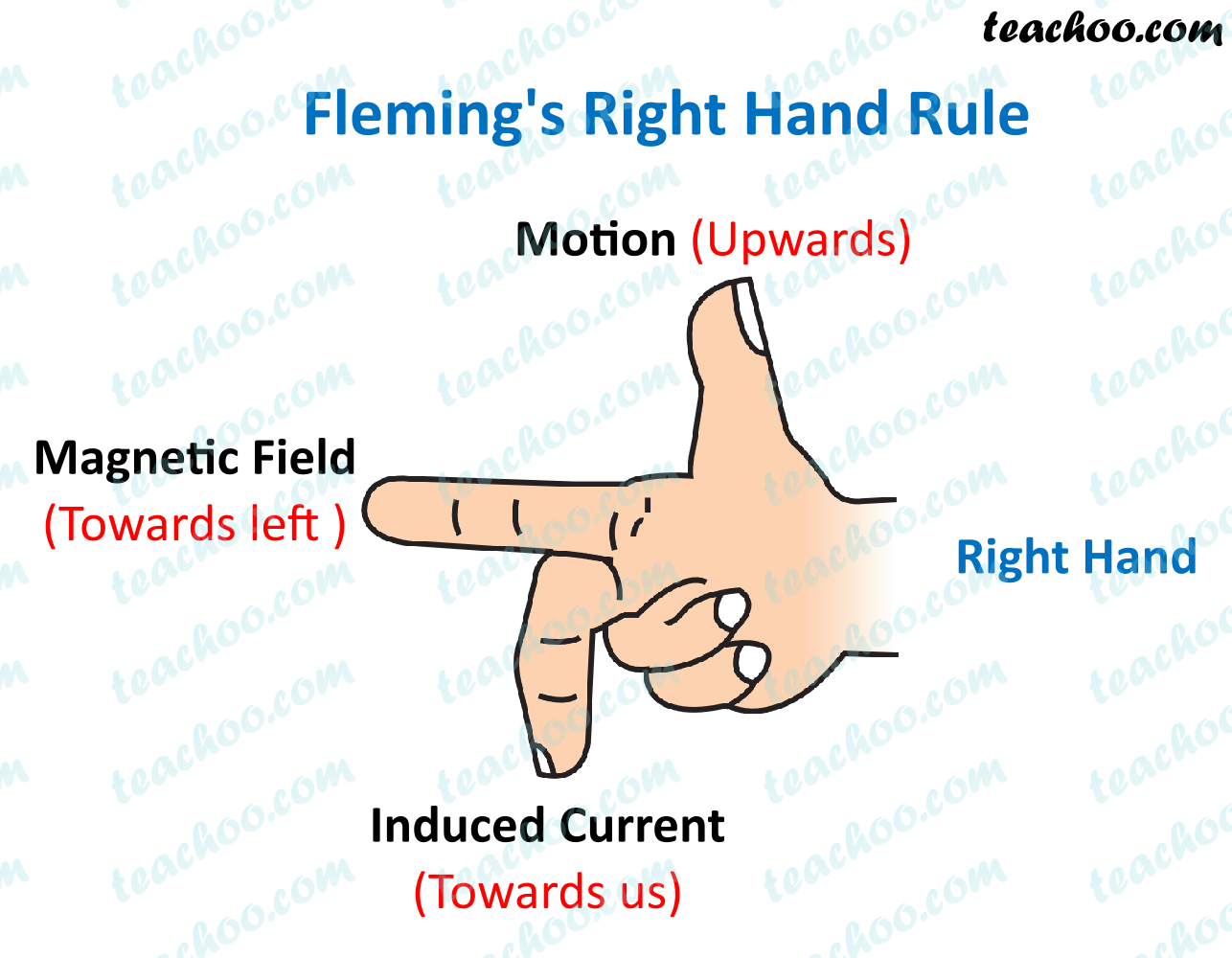Then,

• Our Thumb represents Direction of Motion of Conductor
• Index Finger represents Direction of Magnetic Field
• Middle Finger shows direction of Induced current

To remember this, remember FBI

• F - Force or Motion
• B - Magnetic Field
• I - Induced Current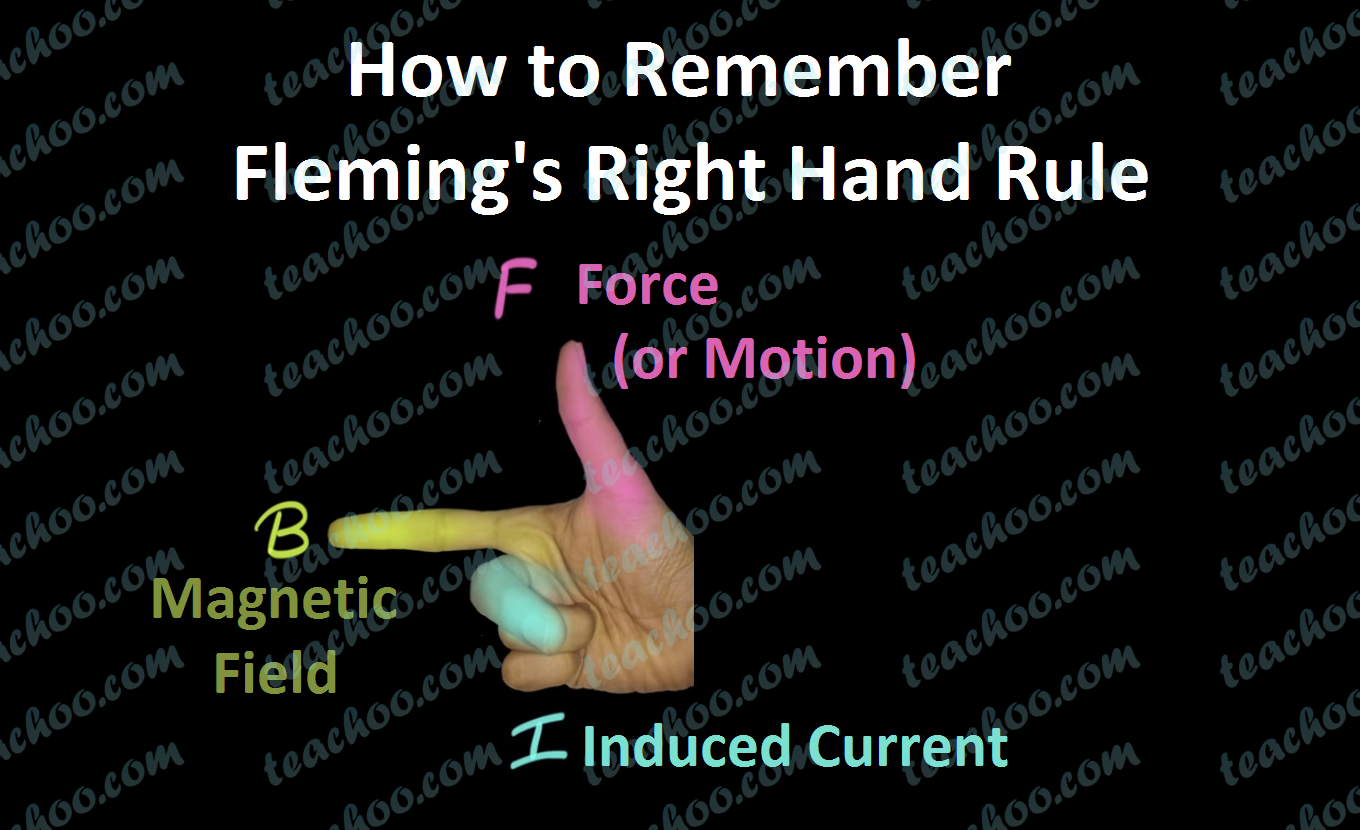Note : FBI is used to remember both Left and Right Rule.

But, always remember... For Induced Current , we use Right Hand Rule

Let's do some questions

## Questions

### What is the direction of induced current in the below figure?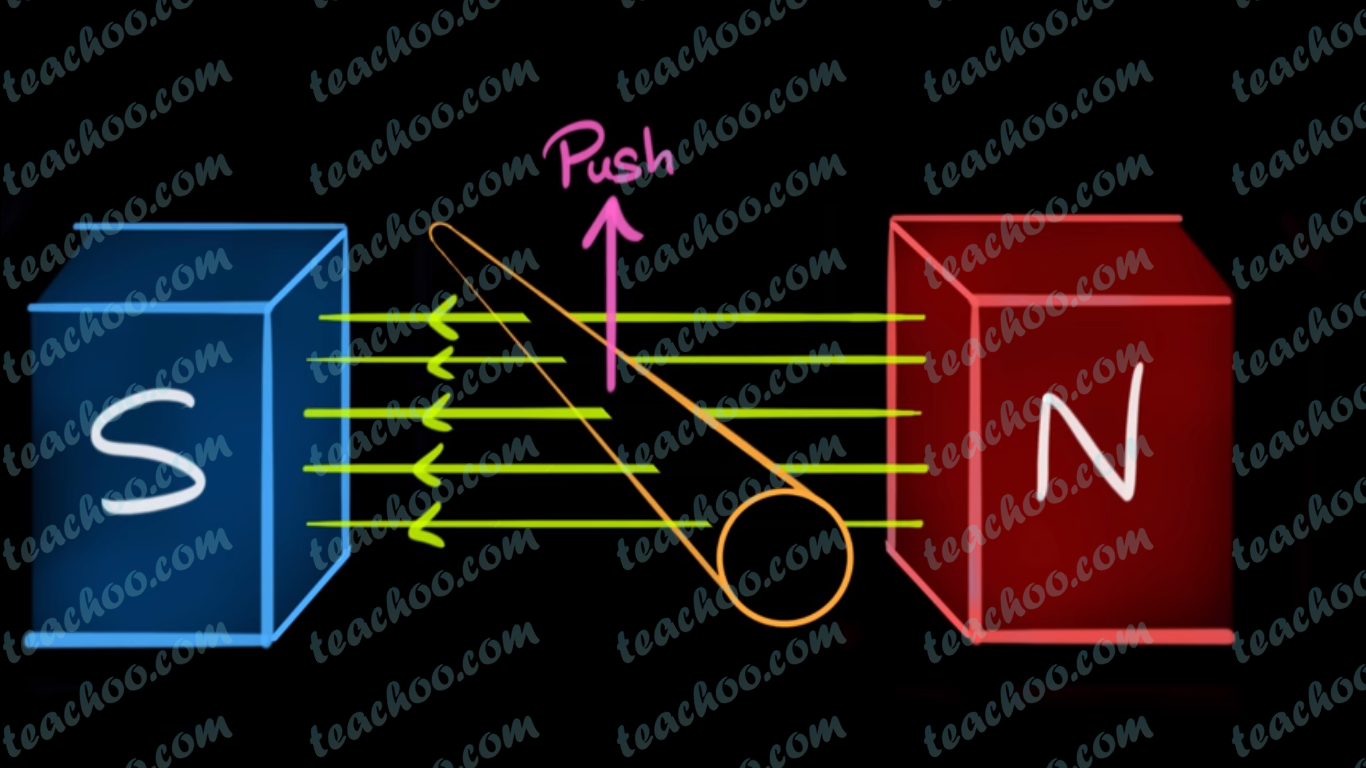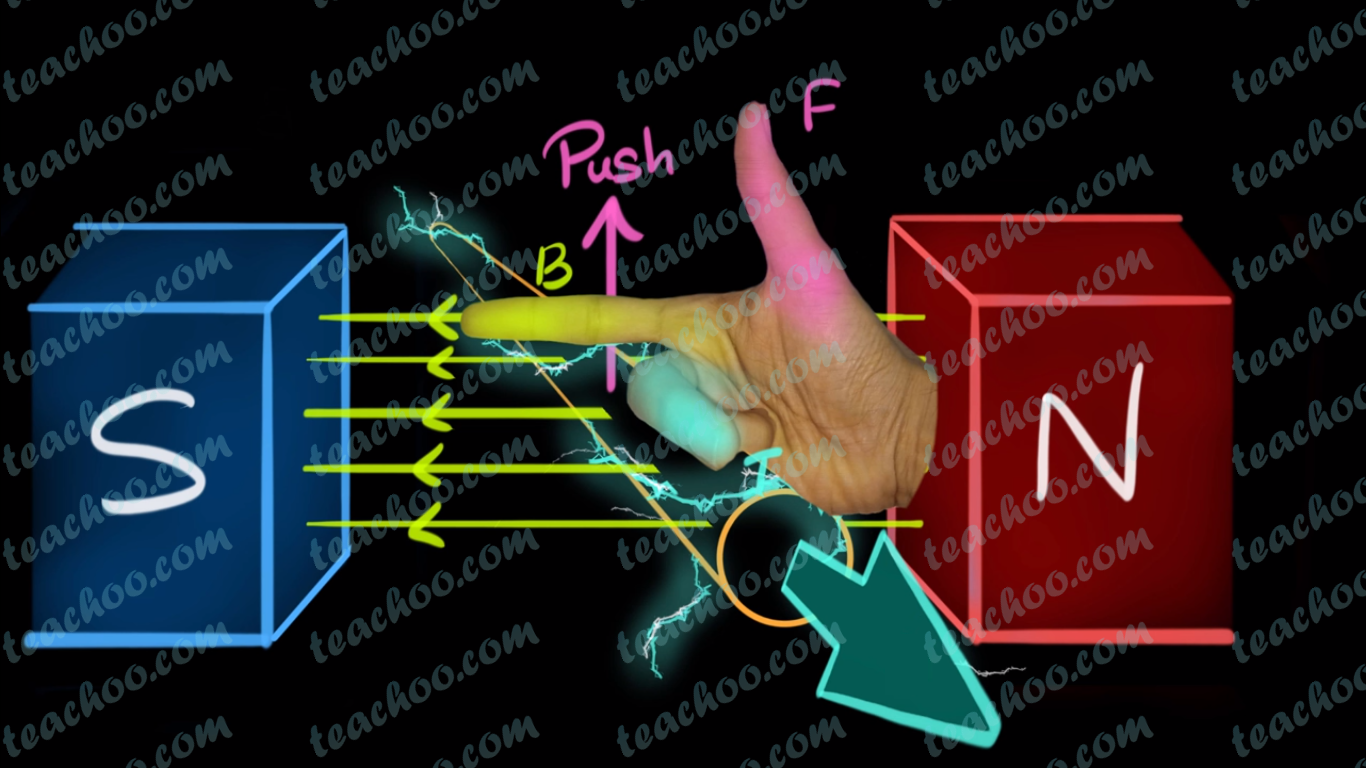### Find Direction of Induced Current in the figure below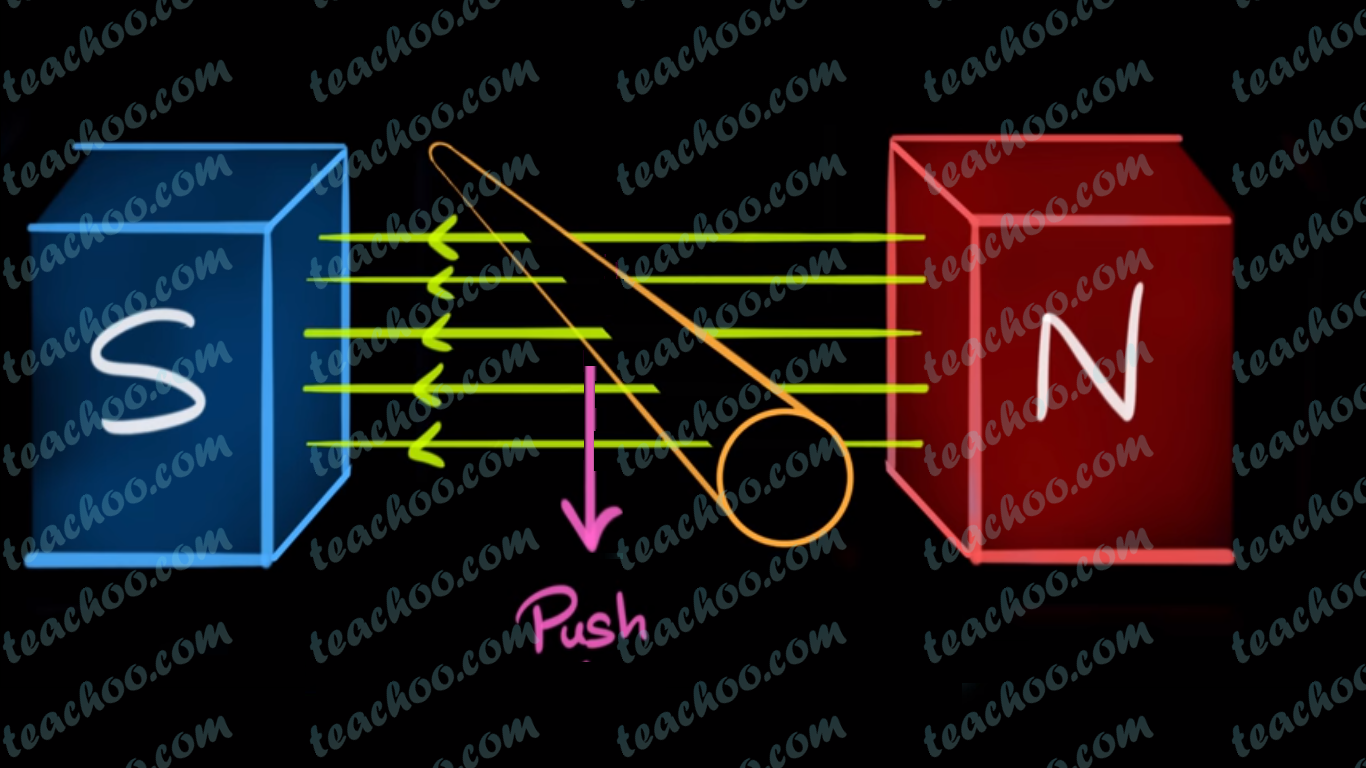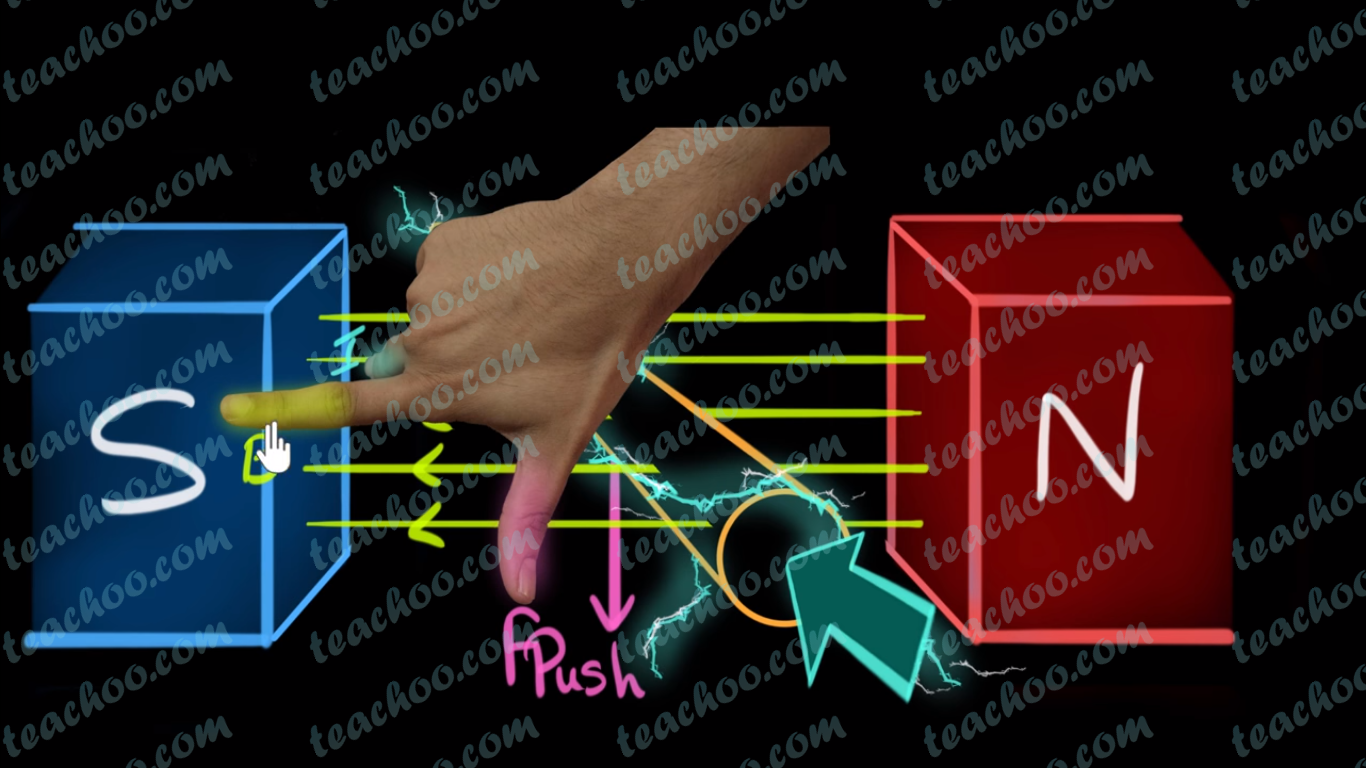### Given Direction of Magnetic Field and Motion. Use Fleming's Right Hand Rule to Find Direction of Induced Current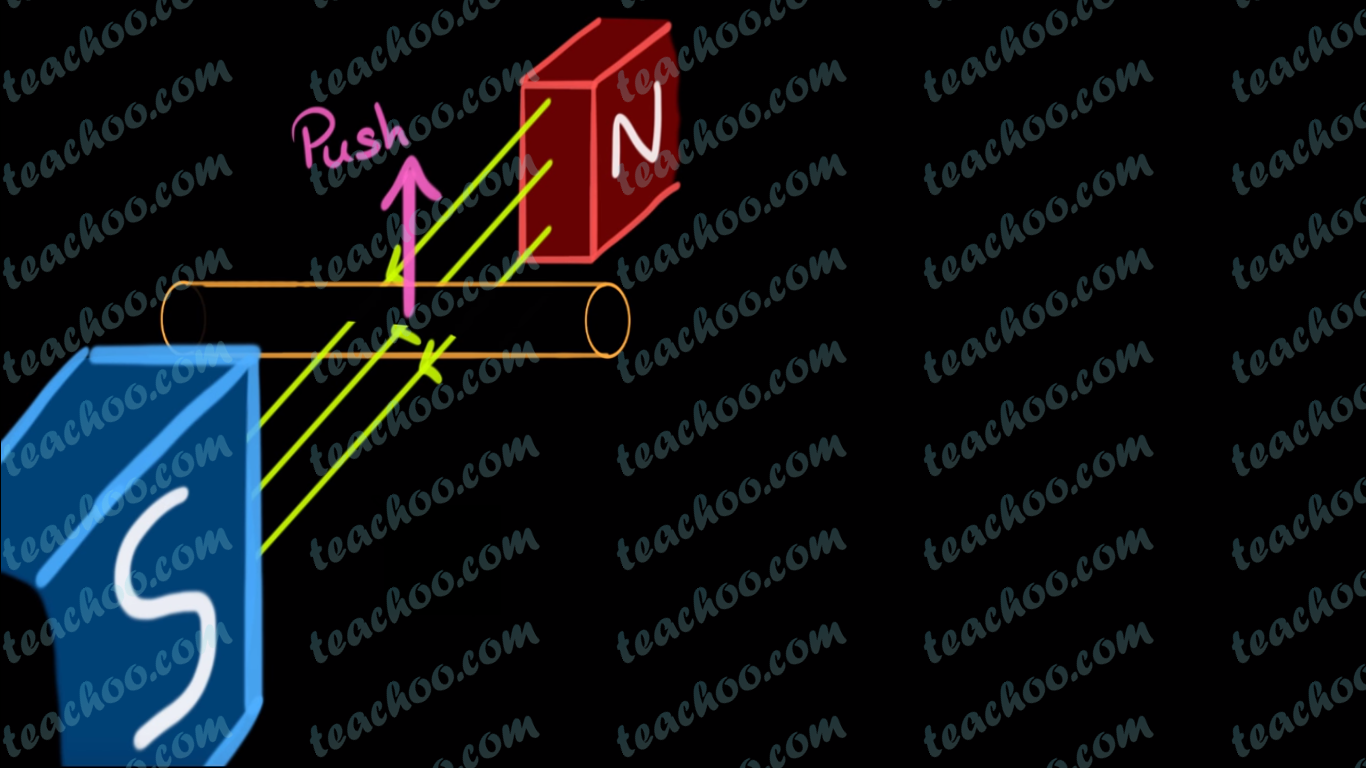(adsbygoogle = window.adsbygoogle || []).push({});